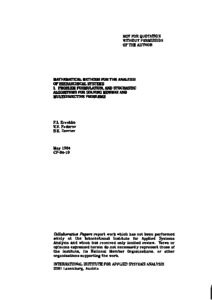# Mathematical Methods for the Analysis of Hierarchical Systems. 1. Problem Formulation, and Stochastic Algorithms for Solving Minimax and Multiobjective Problems

Ereshko, F., Fedorov, V., & Zavriev, S.K. (1984). Mathematical Methods for the Analysis of Hierarchical Systems. 1. Problem Formulation, and Stochastic Algorithms for Solving Minimax and Multiobjective Problems. IIASA Collaborative Paper. IIASA, Laxenburg, Austria: CP-84-019Preview
Text
CP-84-019.pdf

## Abstract

This is the first of two papers dealing with mathematical methods that can be used to analyze hierarchical systems. In this paper, the authors look at the situation that arises when certain decision-making powers are delegated to various elements within a hierarchical structure. It is found that these elements inevitably begin to operate in accordance with their own interests, which are not necessarily those of the system as a whole. Thus we have the problem of how to distribute the decision-making functions between the central body and the other parts of the system in such a way that the efficiency of the control system is maximized with respect to the global criterion.

The authors take a game-theoretical approach to this problem. looking first at two-level hierarchical systems and using Germeyer's games as a model. They derive a number of methods for solving the problem thus formulated, and give some numerical results obtained using two of the resulting algorithms.

Item Type: Monograph (IIASA Collaborative Paper) Interactive Decision Analysis Program (IDA) IIASA Import 15 Jan 2016 01:55 27 Aug 2021 17:12 http://pure.iiasa.ac.at/2558View Item